HEJ, HU ISSN 1418-7108Manuscript no.: ANM-000926-A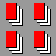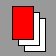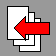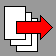# Important properties of prime numbers

Results in the ancient time
THEOREM 3. The number of primes is infinite. PROOF. (By EUCLID) Let us assume, that there are only finite prime numbers,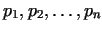. Let us consider the number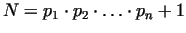. This number is not divisible by the primes listed above, and the number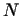is not listed as a prime number, so it cannot be written in the form of Theorem 2. But this is not possible, thusis a new prime itself, or the product of new primes not listed.

> ifactor(2*3*5*7*11*13*17+1);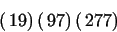PROPOSITION 1. If a number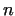has a prime divisor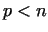, then it has a prime divisor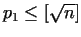.
Using this result, we are able to specify the prime numbers until, if we know the primes until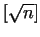. The following method is called the sieve of ERATOSTHENES after the author. Let us write the positive integer numbers beginning with 2 (or 1). Let us consider the first number in the list (we erase and skip the number 1), and erase its multiples. After it we take the following non-erased number in the list, and repeat the procedure. If we reach a non-erased number greater than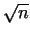, then the sieving is complete.
After these results there was only very limited development in the prime number theory until the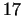th century, FERMAT's time. In the following the most important results of the past four centuries will be summarized.
The number of primes Let us denote the number of prime elements less-equal to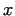by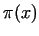, whereis a positive real number. From the result of EUCLID follows, that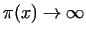, if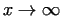. Already EULER noted, that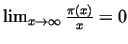. This was first proved by LEGENDRE. The following very important theorem was conjectured in the late 1790s by LEGENDRE and GAUSS, and first proved by HADAMARD and DE LA VALLÉE POUSSIN (1896) with extremely complicated mathematical tools. Elementary proof was given first by PAUL ERDOS and A. SEELBERG (1948).
THEOREM 4. The big prime number theorem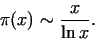REMARKS 1. The notation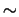means "asymptotic equal" i.e.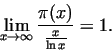2. Let us denote theth prime number by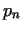. From the result of the theorem follows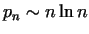. 3. The approach presented in Theorem 4. can be improved with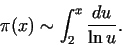To illustrate the results of the theorem with Maple, we write the function, and delineate it with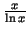in a logarithmic coordinate-system.

> a:=0:
> for i from 3 to 10000 do  a[i]:=a[i-1]:
>   if isprime(i-1) then a[i]:=a[i]+1 fi:
> od:
> pi:=x->if x=trunc(x) then a[x] else a[trunc(x+1)] fi:

> with(plots):
> loglogplot(\{x/ln(x),pi(x)\},x=1.2..500,y=1..200);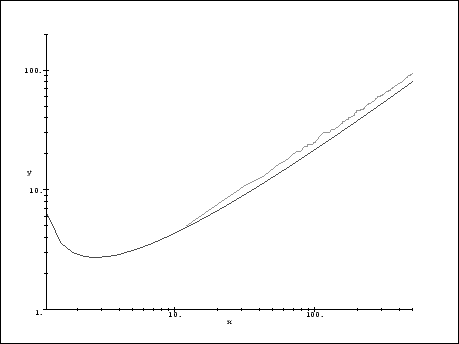By studying the figure we can see the stepfunction-like behaviour of(plotted above), and the fact, that for larger numbers both of the functions fit tight the same asymptotic line. However, between two asymptotic equal functions it is allowed to be very large differences.

> pi(10000); evalf(10000/ln(10000));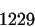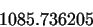> ""/";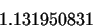To point 2. of the Theorem, we can produce the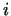th prime number with the function ithprime.

> ithprime(1000), evalf(1000*ln(1000));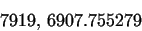THEOREM 5. (First proved by EULER) The sum of the reciprocal value of prime numbers is infinite, i.e.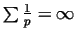.
REMARKS 1. Comparing with the sum of the reciprocal value of square numbers we get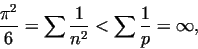so the prime numbers are situated more densely. 2. It is true moreover, that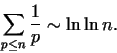Similarly as above, we write the functionsand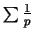to test their behaviour.

> sumsquare:=x->sum(1/n^2,n=1..x);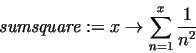> sumprime:=x->sum(1/ithprime(p),p=1..x);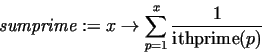> evalf(sumsquare(1000)), evalf(sumprime(1000));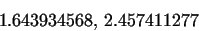The function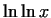grows very slowly.

> evalf(ln(ln(1000))),evalf(ln(ln(1000000)));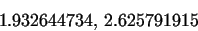The distance of primes Analysing the result of Theorem 4., we conclude that the primes gradually become rarer and rarer (on the average). Their distribution is nevertheless irregular. For example it is obvious, that the sequence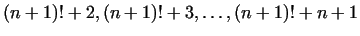contains no primes, at the same time we know very large consecutive primes. Let us denote the difference of theth and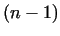th prime number by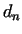, i.e.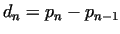. There are very important results for large and small prime differences. From Theorem 4. follows that given an arbitrary small number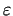there are infinitely many numbersfor which a)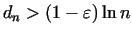, b)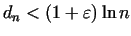. One of the important results for large prime differences was stated by BERTRAND and proved by CSEBISEV, namely ifis a positive integer, then there is always (at least one) prime number betweenand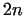. We know moreover, that there exists a constant number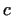, that for every suitable large numbersatisfies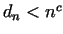, e.g.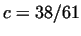can be written. It is an open question, however, that between two consecutive square numbers falls in every case a prime number. If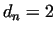, than we call the numbersand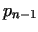twin primes. It is a very old conjecture, that there are infinitely many twin primes. Let us denote the number of twin primes less equal thanby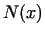. The most important result here is, that there exists a positive constant, for which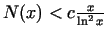. Considering the prime number theorem this means thatis small compared with. To collect observations with Maple about the prime distances we can write programs with the tools reviewed above. Here a simple example will be presented, searching twin primes in a given interval.

> twinprimes:=proc(a,limit)
> local i,prev,act;
>   prev:=isprime(a);
>   for i from a by 2 to a+limit do
>     act:=isprime(i);
>     if prev and act then lprint(i-2,i) fi; prev:=act
>   od
> end:

> twinprimes(10^9+1, 1000);

1000000007    1000000009
1000000409    1000000411
1000000931    1000000933

To find the prime neighbours of an arbitrary number we can use the functions prevprime and nextprime, too.

> b:=12345678901234567890:
> prevprime(b), nextprime(b);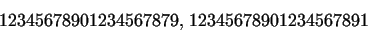HEJ, HU ISSN 1418-7108Manuscript no.: ANM-000926-A